# Designing Lowpass FIR Filters

This example shows how to design lowpass FIR filters. Many of the concepts presented here can be extended to other responses such as highpass, bandpass, etc.

FIR filters are widely used due to the powerful design algorithms that exist for them, their inherent stability when implemented in non-recursive form, the ease with which one can attain linear phase, their simple extensibility to multirate cases, and the ample hardware support that exists for them among other reasons. This example showcases functionality in the DSP System Toolbox™ for the design of lowpass FIR filters with a variety of characteristics.

### Obtaining Lowpass FIR Filter Coefficients

Lowpass Filter Design in MATLAB provides an overview on designing lowpass filters with DSP System Toolbox. To summarize, two functions are presented that return a vector of FIR filter coefficients: `firceqrip` and `firgr`. The `firceqrip` is used when the filter order (equivalently the filter length) is known and fixed.

```N = 100; % FIR filter order Fp = 20e3; % 20 kHz passband-edge frequency Fs = 96e3; % 96 kHz sampling frequency Rp = 0.00057565; % Corresponds to 0.01 dB peak-to-peak ripple Rst = 1e-4; % Corresponds to 80 dB stopband attenuation eqnum = firceqrip(N,Fp/(Fs/2),[Rp Rst],'passedge'); % eqnum = vec of coeffs fvtool(eqnum,'Fs',Fs) % Visualize filter```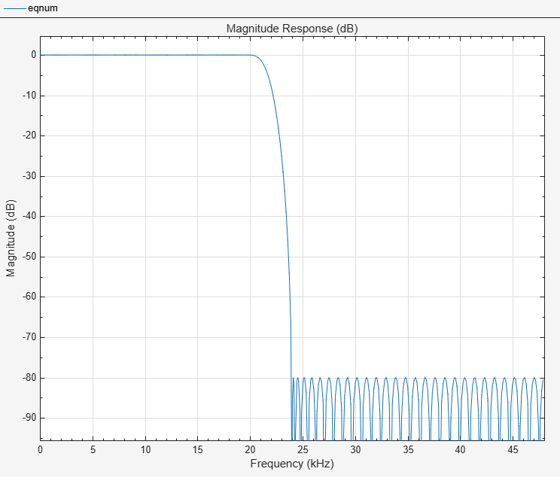The choice of a filter order of 100 was arbitrary. In general, a larger order results in a better approximation to ideal at the expense of a more costly implementation. Doubling the order roughly reduces the filter's transition width in half (assuming all other parameters remain the same).

```N2 = 200; % Change filter order from 100 to 200 eqNum200 = firceqrip(N2,Fp/(Fs/2),[Rp Rst],'passedge'); fvt = fvtool(eqnum,1,eqNum200,1,'Fs',Fs); legend(fvt,"FIR filter, order = "+[N N2])```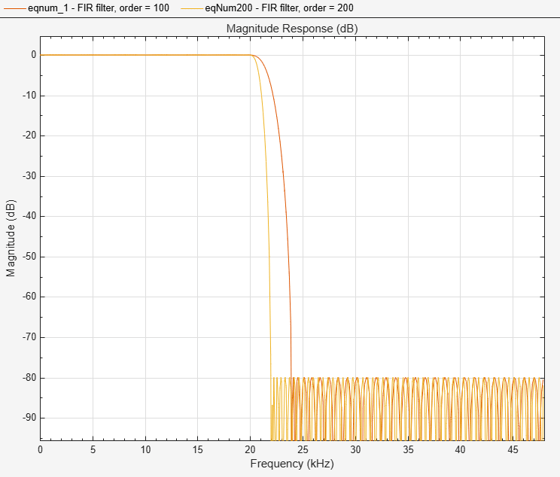### Minimum-Order Lowpass Filter Design

Instead of specifying the filter order, `firgr` can be used to determine the minimum-order required to meet the design specifications. In order to do so, it is necessary to specify the width of the transition region. This is done by setting the stopband edge frequency.

```Fst = 23e3; % Transition Width = Fst - Fp numMinOrder = firgr('minorder',[0,Fp/(Fs/2),Fst/(Fs/2),1],[1 1 0 0],... [Rp Rst]); fvt = fvtool(eqnum,1,eqNum200,1,numMinOrder,1,'Fs',Fs); legend(fvt,"FIR filter, order = "+[N N2 numel(numMinOrder)])```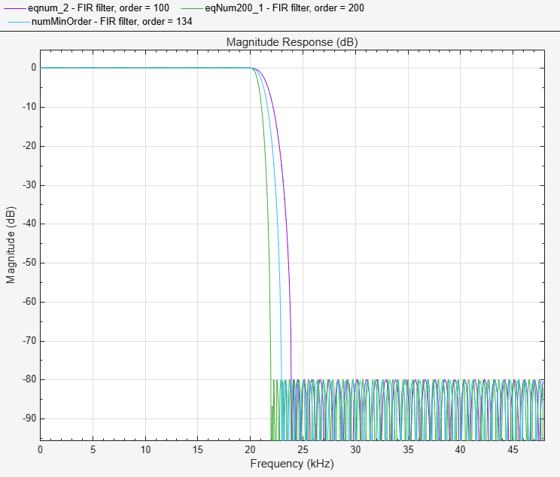It is also possible to design filters of minimum even order ('mineven') or minimum odd order ('minodd') through the `firgr` function.

### Implementing the Lowpass FIR Filter

Once the filter coefficients have been obtained, the filter can be implemented with `dsp.FIRFilter`. This supports double/single precision floating-point data as well as fixed-point data. It also supports C and HDL code generation as well as optimized code generation for ARM® Cortex® M and ARM Cortex A.

```lowpassFIR = dsp.FIRFilter('Numerator',eqnum); %or eqNum200 or numMinOrder fvtool(lowpassFIR,'Fs',Fs)```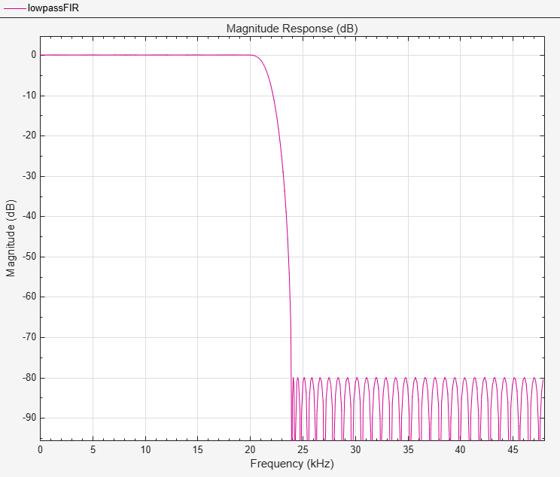In order to perform the actual filtering, call the FIR directly like a function. The following code filters Gaussian white noise and shows the resulting filtered signal in a spectrum analyzer for 10 seconds.

```scope = spectrumAnalyzer('SampleRate',Fs,'AveragingMethod','exponential','ForgettingFactor',0.5); show(scope); tic while toc < 10 x = randn(256,1); y = lowpassFIR(x); scope(y); end```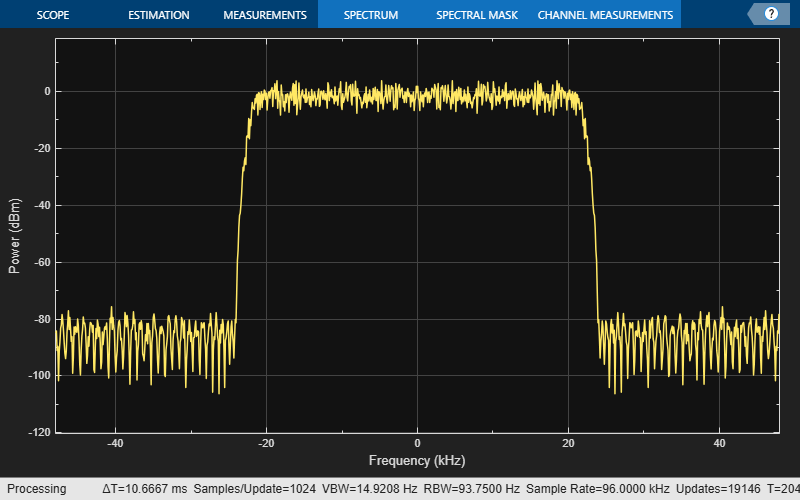### Designing and Implementing the Filter in One Step

As a convenience, designing and implementing the filter can be done in a single step using `dsp.LowpassFilter`. This also supports floating-point, fixed-point, C code generation, and ARM Cortex M and ARM Cortex A optimizations.

```lowpassFilt = dsp.LowpassFilter('DesignForMinimumOrder',false, ... 'FilterOrder',N,'PassbandFrequency',Fp,'SampleRate',Fs,... 'PassbandRipple',0.01, 'StopbandAttenuation',80); tic while toc < 10 x = randn(256,1); y = lowpassFilt(x); scope(y); end```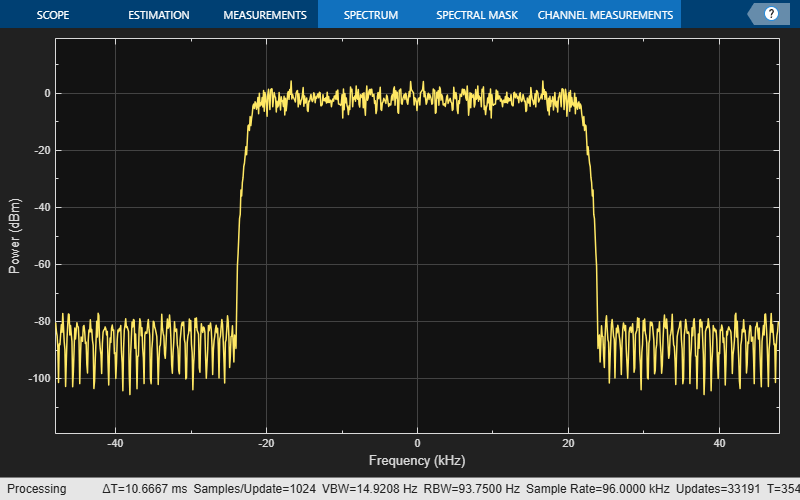Notice that as a convenience, specifications are entered directly using dB values. The passband ripple can be examined by selecting the "View" menu in FVTool and then selecting "Passband". `dsp.LowpassFilter` can also be used for IIR (biquad) designs.

`fvtool(lowpassFilt,'Fs',Fs)`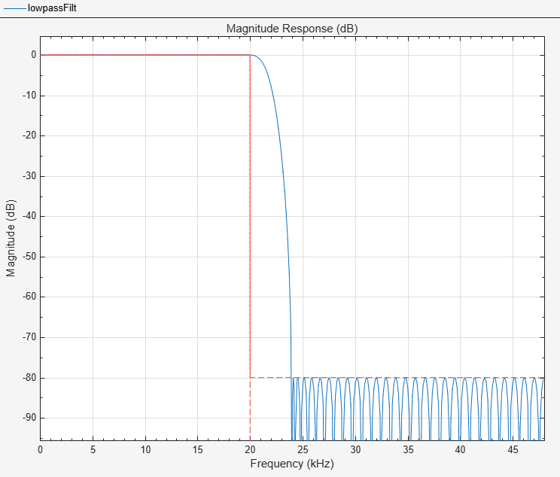### Obtaining the Filter Coefficients

The filter coefficients can be extracted from `dsp.LowpassFilter` by using the `tf` function.

`eqnum = tf(lowpassFilt);`

### Tunable Lowpass FIR Filters

Lowpass FIR filters in which the cutoff frequency can be tuned at run-time can be implemented using the '`dsp.VariableBandwidthFIRFilter`' object. These filters do not provide the same granularity of control over the filter's response characteristic, but they do allow for dynamic frequency response.

```vbwFilter = dsp.VariableBandwidthFIRFilter('CutoffFrequency',1e3); tic told = 0; while toc < 10 t = toc; if floor(t) > told % Add 1 kHz every second vbwFilter.CutoffFrequency = vbwFilter.CutoffFrequency + 1e3; end x = randn(256,1); y = vbwFilter(x); scope(y); told = floor(t); end```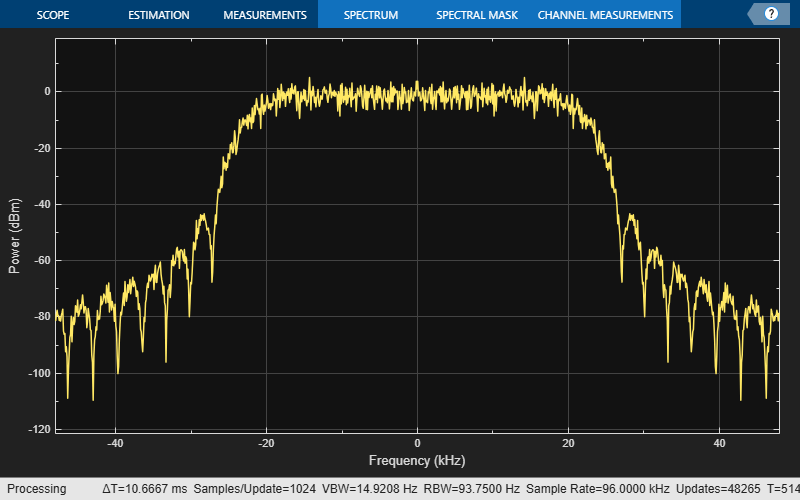### Advanced Design Options: Optimal Non-Equiripple Lowpass Filters

So far all designs used have been optimal equiripple designs. Equiripple designs achieve optimality by distributing the deviation from the ideal response uniformly. This has the advantage of minimizing the maximum deviation (ripple). However, the overall deviation, measured in terms of its energy tends to be large. This may not always be desirable. When lowpass filtering a signal, this implies that remnant energy of the signal in the stopband may be relatively large. When this is a concern, least-squares methods provide optimal designs that minimize the energy in the stopband. `fdesign.lowpass` can be used to design least-squares and other kinds of lowpass filters. The following code compares a least-squares FIR design to an FIR equiripple design with the same filter order and transition width:

```lowpassSpec = fdesign.lowpass('N,Fp,Fst',133,Fp,Fst,Fs); lsFIR = design(lowpassSpec,'firls','SystemObject',true); LP_MIN = dsp.FIRFilter('Numerator',numMinOrder); fvt = fvtool(LP_MIN,lsFIR,'Fs',Fs); legend(fvt,'Equiripple design','Least-squares design')```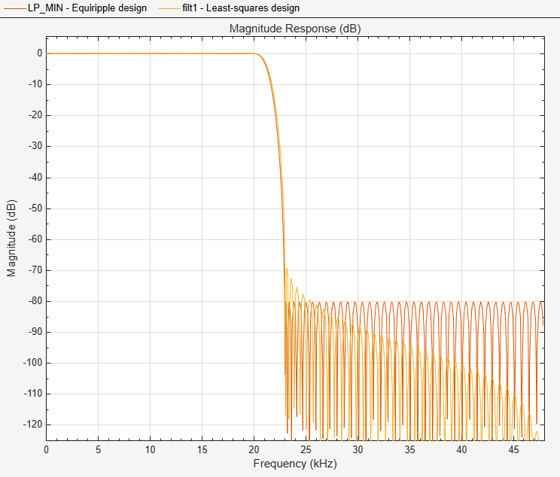Notice how the attenuation in the stopband increases with frequency for the least-squares designs while it remains constant for the equiripple design. The increased attenuation in the least-squares case minimizes the energy in that band of the signal to be filtered.

### Equiripple Designs with Increasing Stopband Attenuation

An often undesirable effect of least-squares designs is that the ripple in the passband region close to the passband edge tends to be large. For lowpass filters in general, it is desirable that passband frequencies of a signal to be filtered are affected as little as possible. To this extent, an equiripple passband is generally preferable. If it is still desirable to have an increasing attenuation in the stopband, equiripple design options provide a way to achieve this.

```FIR_eqrip_slope = design(lowpassSpec,'equiripple','StopbandShape','1/f',... 'StopbandDecay',4,'SystemObject',true); fvt = fvtool(lsFIR,FIR_eqrip_slope,'Fs',Fs); legend(fvt,'Least-squares design',... 'Equiripple design with stopband decaying as (1/f)^4')```Notice that the stopbands are quite similar. However the equiripple design has a significantly smaller passband ripple in the vicinity of the passband-edge frequency, 20 kHz:

```mls = measure(lsFIR); meq = measure(FIR_eqrip_slope); mls.Apass```
```ans = 0.0121 ```
`meq.Apass`
```ans = 0.0046 ```

Yet another possibility is to use an arbitrary magnitude specification and select two bands (one for the passband and one for the stopband). Then, by using weights for the second band, it is possible to increase the attenuation throughout the band. For more information on this and other arbitrary magnitude designs see Arbitrary Magnitude Filter Design.

```B = 2; % Number of bands F = [0 Fp linspace(Fst,Fs/2,40)]; A = [1 1 zeros(1,length(F)-2)]; W = linspace(1,100,length(F)-2); lowpassArbSpec = fdesign.arbmag('N,B,F,A',N,B,F(1:2),A(1:2),F(3:end), ... A(3:end),Fs); lpfilter = design(lowpassArbSpec,'equiripple','B2Weights',W, ... 'SystemObject',true); fvtool(lpfilter,'Fs',Fs);```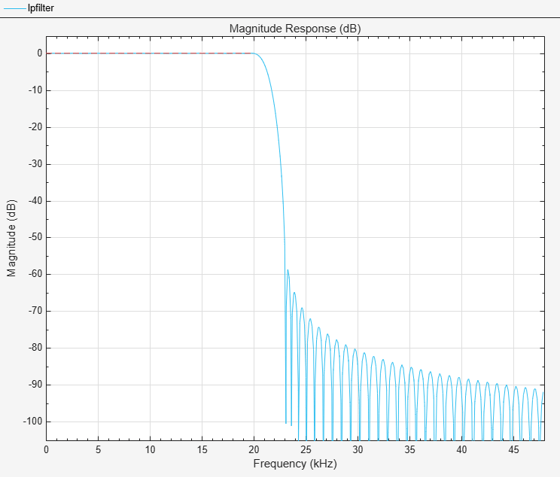### Minimum-Phase Lowpass Filter Design

So far, we have only considered linear-phase designs. Linear phase is desirable in many applications. Nevertheless, if linear phase is not a requirement, minimum-phase designs can provide significant improvements over linear phase counterparts. However, minimum-phase designs are not always numerically robust. Always check the design with FVTool.

As an example of the advantages of minimum-phase designs, consider the comparison of a linear-phase design with a minimum-phase design that meets the same design specifications:

```Fp = 20e3; Fst = 22e3; Fs = 96e3; Ap = 0.06; Ast = 80; lowpassSpec = fdesign.lowpass('Fp,Fst,Ap,Ast',Fp,Fst,Ap,Ast,Fs); linphaseSpec = design(lowpassSpec,'equiripple','SystemObject',true); eqripSpec = design(lowpassSpec,'equiripple','minphase',true,... 'SystemObject',true); fvt = fvtool(linphaseSpec,eqripSpec,'Fs',Fs); legend(fvt,... 'Linear-phase equiripple design',... 'Minimum-phase equiripple design')```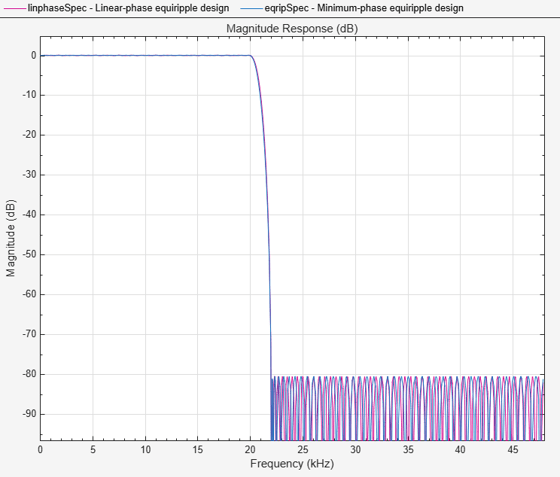Notice that the number of coefficients has been reduced from 173 to 141. The group-delay plot also reveals advantages of the minimum-phase design. Notice how the group-delay is much smaller (in particular in the passband region).

```fvt = fvtool(linphaseSpec,eqripSpec,'Fs',Fs,... 'Analysis','grpdelay'); legend(fvt,... 'Linear-phase equiripple design',... 'Minimum-phase equiripple design')```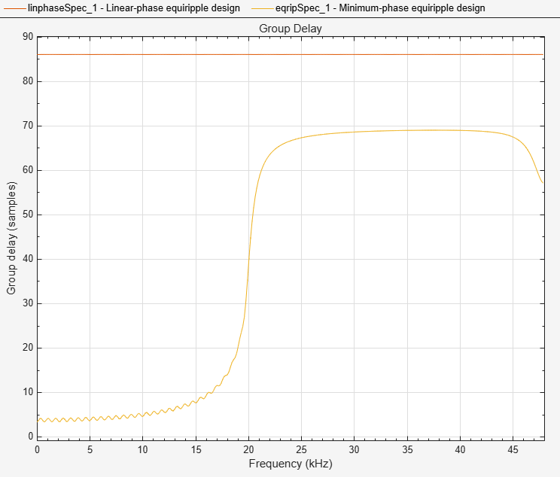### Minimum-Order Lowpass Filter Design Using Multistage Techniques

A different approach to minimizing the number of coefficients that does not involve minimum-phase designs is to use multistage techniques. Here we show an interpolated FIR (IFIR) approach. This approach breaks down the design problem into designing two filters in cascade. For this example, the design requires 151 coefficients rather than 173. For more information on this, see Efficient Narrow Transition-Band FIR Filter Design.

```Fp = 20e3; Fst = 22e3; Fs = 96e3; Ap = 0.06; Ast = 80; lowpassSpec = fdesign.lowpass('Fp,Fst,Ap,Ast',Fp,Fst,Ap,Ast,Fs); interpFilter = design(lowpassSpec,'ifir','SystemObject',true); cost(interpFilter)```
```ans = struct with fields: NumCoefficients: 151 NumStates: 238 MultiplicationsPerInputSample: 151 AdditionsPerInputSample: 149 ```
```fvt = fvtool(linphaseSpec,interpFilter,'Fs',Fs); legend(fvt,... 'Linear-phase equiripple design',... 'Interpolated FIR equiripple design (two stages)')```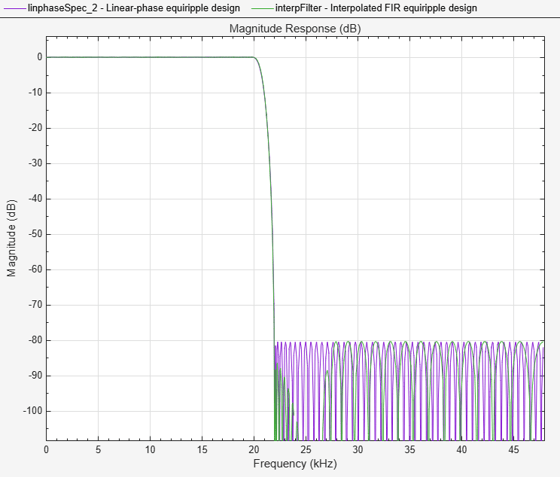In this case, the group-delay plot reveals disadvantages of the IFIR design. While IFIR designs do provide linear phase, the group delay is in general larger than a comparable single-stage design.

```fvt = fvtool(linphaseSpec,interpFilter,'Fs',Fs,'Analysis','grpdelay'); legend(fvt,... 'Linear-phase equiripple design',... 'Interpolated FIR equiripple design (two stages)')```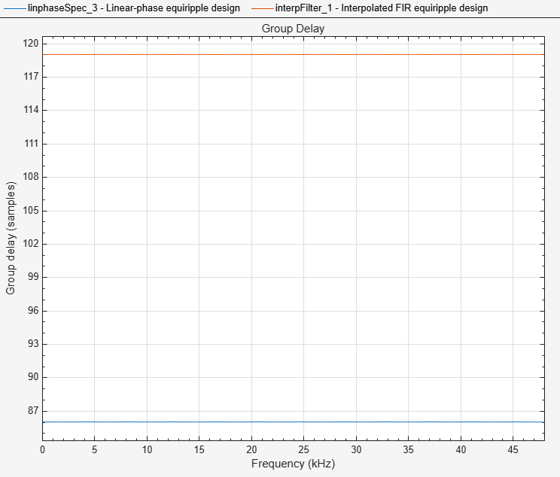### Lowpass Filter Design for Multirate Applications

Lowpass filters are extensively used in the design of decimators and interpolators. For more information, see Design of Decimators and Interpolators. For more information on multistage techniques that result in very efficient implementations, see Multistage Rate Conversion.